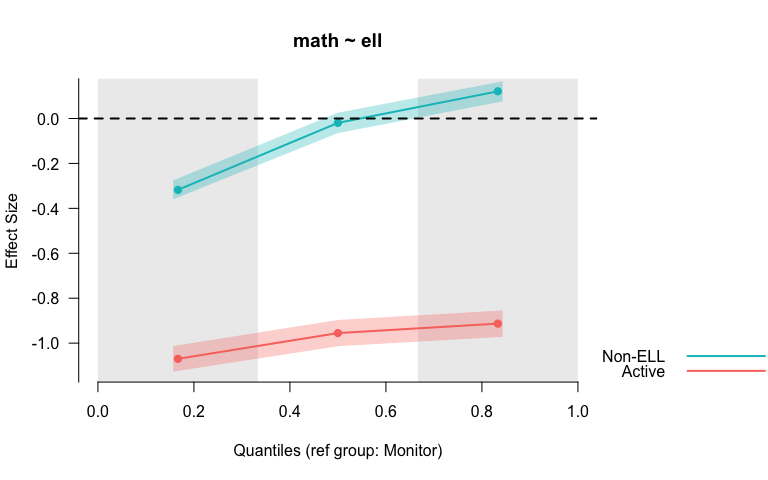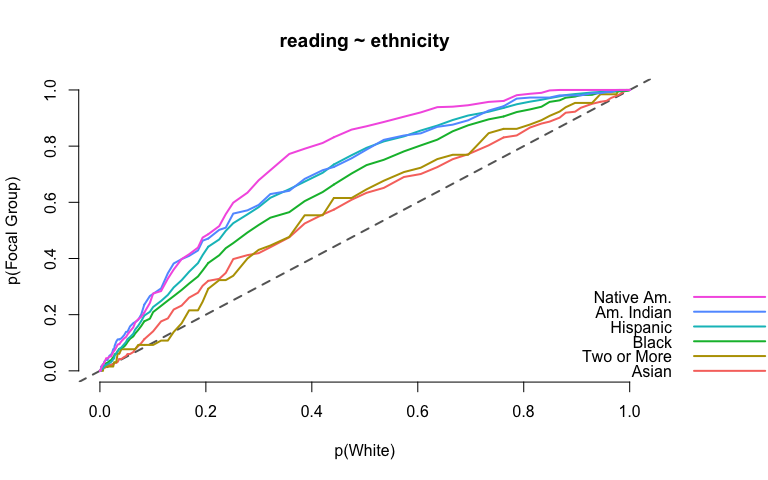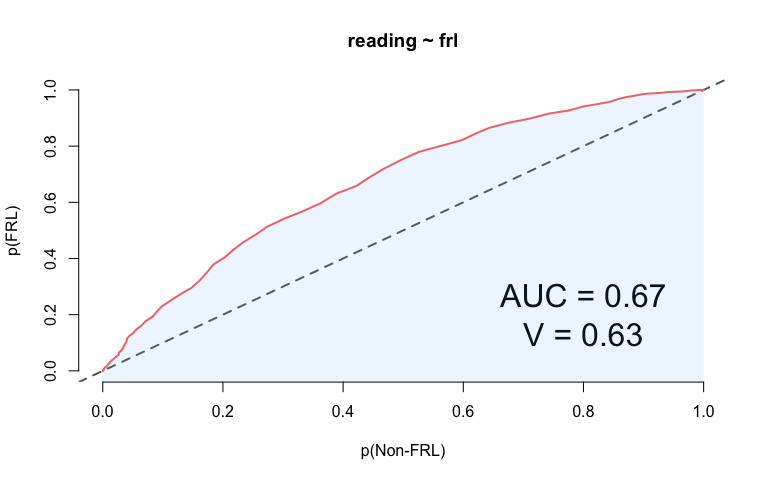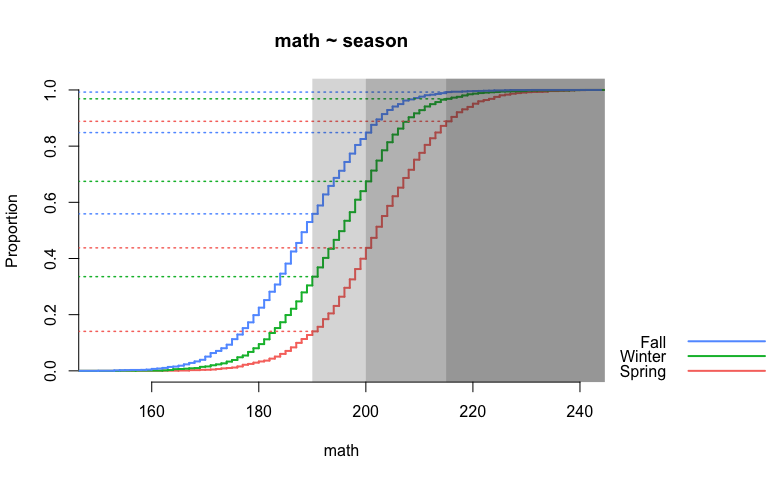# Visualization and Estimation of Effect Sizes

A variety of methods are provided to estimate and visualize distributional differences in terms of effect sizes. Particular emphasis is upon evaluating differences between two or more distributions across the entire scale, rather than at a single point (e.g., differences in means). For example, Probability-Probability (PP) plots display the difference between two or more distributions, matched by their empirical CDFs (see Ho and Reardon, 2012; ), allowing for examinations of where on the scale distributional differences are largest or smallest. The area under the PP curve (AUC) is an effect-size metric, corresponding to the probability that a randomly selected observation from the x-axis distribution will have a higher value than a randomly selected observation from the y-axis distribution. Binned effect size plots are also available, in which the distributions are split into bins (set by the user) and separate effect sizes (Cohen's d) are produced for each bin - again providing a means to evaluate the consistency (or lack thereof) of the difference between two or more distributions at different points on the scale. Evaluation of empirical CDFs is also provided, with built-in arguments for providing annotations to help evaluate distributional differences at specific points (e.g., semi-transparent shading). All function take a consistent argument structure. Calculation of specific effect sizes is also possible. The following effect sizes are estimable: (a) Cohen's d, (b) Hedges' g, (c) percentage above a cut, (d) transformed (normalized) percentage above a cut, (e) area under the PP curve, and (f) the V statistic (see Ho, 2009; ), which essentially transforms the area under the curve to standard deviation units. By default, effect sizes are calculated for all possible pairwise comparisons, but a reference group (distribution) can be specified.

R Package for effect size visualizations.

This package is designed to visually compare two or more distributions across the entirety of the scale, rather than only by measures of central tendency (e.g., means). There are also some functions for estimating effect size, including Cohen's d, Hedges' g, percentage above a cut, transformed (normalized) percentage above a cut, the area under the curve (conceptually equivalent to the probability that a randomly selected individual from Distribution A has a higher value than a randomly selected individual from Distribution B), and the V statistic, which essentially transforms the area under the curve to standard deviation units (see Ho, 2009).

## Installation

Install directly from CRAN with

Or the development version from from github with:

## Plotting methods

There are three primary data visualizations: (a) binned effect size plots, (b)probability-probability plots, and (c) empirical cumulative distribution functions. All plots should be fully manipulable with calls to the base plotting functions.

At present, the binned effect size plot can only be produced with Cohen's d, although future development will allow the user to select the type of effect size. The binned effect size plot splits the distribution into quantiles specified by the user (defaults to lower, middle, and upper thirds), calculates the mean difference between groups within each quantile bin, and produces an effect size for each bin by dividing by the overall pooled standard deviation (i.e., not by quantile). For exampleNote that in this plot one can clearly see that the magnitude of the differences between the two three groups depends upon scale location (i.e., low achieving students versus average or high achieving students). Both the reference group and the quantiles used can be changed. For example `binned_plot(math ~ ell, benchmarks, ref_group = "Non-ELL", qtiles = seq(0, 1, .2))` would produce the same plot but binned by quintiles, with students who did not receive English language services (Non-ELL) as the reference group.

A probability-probability plot can be produced with a call to `pp_plot` and an equivalent argument structure. In this case, we're visualizing the difference in reading achievement by race/ethnicity. By default, the distribution with the highest mean serves as the reference group, in this case students identifying as White.If the grouping factor has only two levels, the area under the PP curve will be shaded, with the AUC an V statistics annotated onto the plot.The shading and annotations are optional and can be removed. The colors and all other plot features are also fully customizable.

Finally, the `ecdf_plot` function essentially dresses up the base `plot.ecdf` function, but also adds some nice referencing features through additional, optional arguments. Below, I have included the optional `hor_ref = TRUE` argument such that horizontal reference lines appear, relative to the cuts provided.## Estimation Methods

Compute effect sizes for all possible pairwise comparisons.

Or specify a reference group

Other effect sizes are estimated equivalently. For example, compute V (Ho, 2009) with

or AUC with

# esvis 0.2.0.0000

This release is mostly about reformatting code and minor bug fixes. A few changes:

• The viridisLite package is now listed as a `suggests`, and there are options for the plots to be produced with these color schemes, if the package is installed.

• A few of the effect sizes were reversed in 0.1, relative to the focal/reference groups. Those have been fixed.

• There is now a `theme` function that is extensible and allows for custom themes, rather than just the "standard" and "dark" themes.

# Reference manual

install.packages("esvis")

0.3.1 by Daniel Anderson, a year ago

https://github.com/datalorax/esvis

Report a bug at https://github.com/datalorax/esvis/issues

Browse source code at https://github.com/cran/esvis

Authors: Daniel Anderson [aut, cre]

Documentation:   PDF Manual